# Editing event #5100 (stability increased)

#### EightDeer

##### Major
•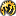•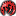•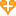•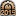•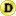•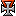•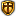•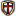•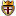•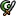•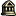•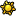•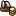•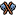•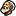•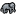•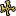•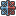•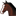•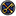•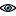•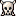•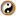•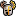•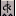•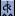I'm trying to make the realm ruler's stability value change the MTTH of event #5100; the intention is that vassals would be affected by the state of the liege's realm.

Two questions about the code at the end of this post: Will this affect independent rulers, and if so how can I make it apply only to vassals?

The code:
Code:
``````		modifier = {
condition = { type = realm_ruler
condition = { type = stability value = 1 }
condition = { type = not value = { type = stability value = 2 } }
}
factor = 0.9
}
modifier = {
condition = { type = realm_ruler
condition = { type = stability value = 2 }
condition = { type = not value = { type = stability value = 3 } }
}
factor = 0.8
}
modifier = {
condition = { type = realm_ruler
condition = { type = stability value = 3 }
}
factor = 0.7
}
modifier = {
condition = { type = realm_ruler
condition = { type = stability value = -1 }
condition = { type = not value = { type = stability value = -2 } }
}
factor = 1.1
}
modifier = {
condition = { type = realm_ruler
condition = { type = stability value = -2 }
condition = { type = not value = { type = stability value = -3 } }
}
factor = 1.2
}
modifier = {
condition = { type = realm_ruler
condition = { type = stability value = -3 }
}
factor = 1.3
}``````

Last edited:

#### jordarkelf

##### 01_TITLE_STRING
•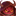•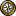•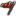•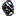•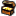•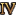•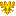•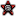•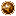•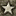•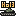•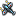•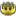•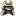•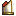•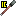••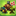•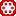•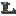•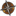•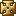•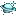•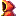•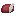•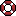••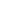••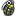•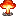•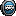•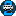•••••••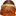•••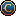•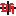•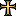••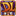•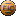•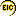•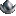Realm_ruler applies to the realm overlord, so either a vassal's lord or an independent ruler himself. It will never match a vassal.

To directly target vassals, use condition = { type = is_vassal }